Refer to our Texas Go Math Grade 6 Answer Key Pdf to score good marks in the exams. Test yourself by practicing the problems from Texas Go Math Grade 6 Module 5 Answer Key Adding and Subtracting integers.

Write an integer to represent each situation.

Question 1.
an elevator ride down 27 stories
First, you need to decide whether the integer is positive or negative
ride down → it would be negative
So, unknown integer is – 27 (minus is for negative and 27 is given number).

Question 2.
a \$ 700 profit
First, you need to decide whether the integer is positive or negative
Profit → it would be positive
So, unknown integer is 700 (it is positive 700 is given number).

46 degrees below zero
First, you need to decide whether the integer is positive or negative
below zero → it would be negative
So, unknown integer is – 46 (minus is for negative and 46 is the given number).

Question 4.
a gain of 12 yards
First, you need to decide whether the integer is positive or negative
gain → it would be positive.
So, unknown integer is 12 (it is positive and 12 is given number).

Find the sum or difference.

Question 5.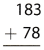3 + 8 = 11
Now, 11 ones equals 1 ten plus 1.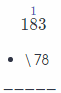8 + 7 + 1 = 16
Now, 16 tens equals 1 hundred plus 6 tens.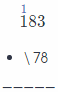1 + 0 + 1 = 2 (last 1 is hundred from adding tens)
Finally, write 2 for hundreds: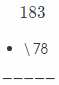Final result is 261.

First add ones, then tens and then hundreds Result is 261.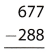First, Subtract ones.
Note that 7 < 8, so regroup 1 ten as 10 ones
7 ones + 10 ones = 17 ones (and 6 tens left)
Now, 17 – 8 = 9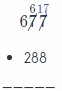Then, Subtract tens.
Note that 6 < 8, so regroup 1 hundred as 10 tens.
6 ones + 10 ones = 16 ones
Now, 16 – 8 = 8: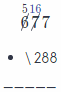Then, subtract hundreds. Because 5 > 2 only subtract them:
5 – 2 = 3
Finally: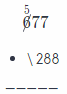Final result is 389.

First add ones, then tens and then hundreds Result is 389.

Question 7.8 + 2 = 10
Now, 10 ones equals 1 ten plus 0.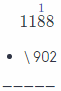8 + 0 + 1 = 0 (1 is ten from adding ones)
Now, write 9 for tens.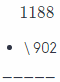1 + 9 = 10
Now, 10 hundreds equals 1 thousand plus 0 hundred1 + 0 + 1 = 2 (1 is thousand from adding hundreds)

Now, write 2 for thousand: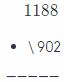Final result is 2090.

First add ones, then tens, then hundreds and finally thousands Result is 2090.

Question 8.First, Subtract ones. Because 7 > 5 only subtract them:
7 – 5 = 2
Now: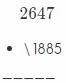Then, subtract tens
Note that 4 < 8, so regroup 1 hundred as 10 tens
4 ones + 10 ones = 14 ones (and 5 hundreds left)
Now, 14 – 8 = 6: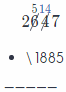Then, subtract hundreds.
Note that 5 < 8, so regroup 1 thousand as 10 hundreds.
5 hundreds + 10 hundreds = 15 hundreds (and 1 thousand left)
Now, 15 – 8 = 7First, subtract thousands:
1 – 1 = 0
Now:Final result is 762.

First subtract ones, then tens, then hundreds and then thousands.
Regroup if necessary. Result is 762.

Graph each number on the number line.Question 9.
7
First, graph number line with units.
To graph 7 start at 0 and move 7 units to the right.

Graph: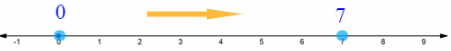– 4
First, graph the number line with units.
To graph – 4 start at 0 and move 4 units to the left.

Graph:Question 11.
– 9
First, graph number line with units.
To graph – 9 start at 0 and move 9 units to the left.

Graph: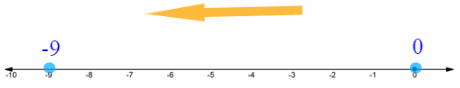Question 12.
4
First, graph number line with units.
To graph 4 start at 0 and move 4 units to the right.

Graph:Visualize Vocabulary
Use the ✓ words to fill in the ovals on the graphic. You may put more than one word in each oval.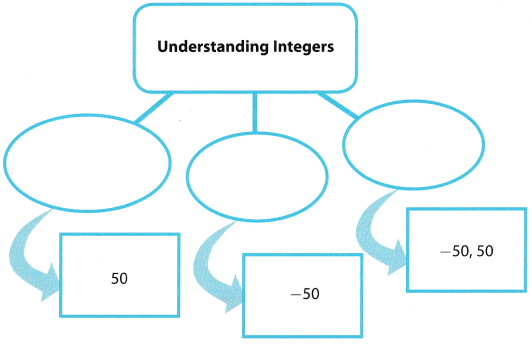Understand Vocabulary

Complete the sentences using the preview words.

Question 1.
The ____________________ of a number gives its distance from zero.
This is because:
|4| = 4, which is its distance from 0.
|- 9| = 9, which is its distance from 0.